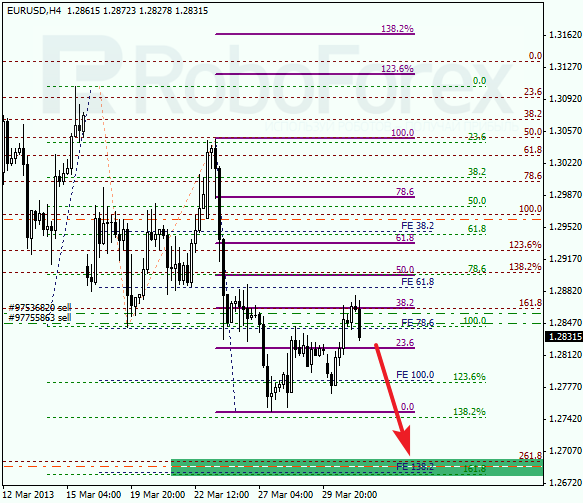Fibonacci Retracements Analysis - Page 1191. Fibonacci Retracements AnalysisThe EUR/USD currency pair rebounded from the level of 38.2%, just as we expected. The bears are trying to renew a descending trend; I opened one more sell order. In the near term, the pair is expected to continue falling down towards the target area, where there are several fibo-levels at the same time.2. Fibonacci Retracements Analysis 30.04.2019 (EURUSD, USDJPY)As we can see in the H4 chart, after breaking the previous low, EURUSD has started a short-term pullback. The pair may start a new descending impulse after it breaks the current low at 1.1110. The downside targets are inside the post-correctional extension area between the retracements of 138.2% and 161.8% at 1.1067 and 1.1002 respectively. The key resistance level is at 1.1324.3. Fibonacci Retracements Analysis 01.05.2019 (GBPUSD, EURJPY)As we can see in the H4 chart, the convergence made GBPUSD start a new correctional uptrend, which has already reached the retracement of 38.2%. The next upside targets may be the retracements of 50.0% and 61.8% at 1.3088 and 1.3141 respectively. If the price breaks the low at 1.2865, the instrument may continue falling towards the long-term retracement of 61.8% at 1.2773.4. Fibonacci Retracements Analysis 02.05.2019 (AUDUSD, USDCAD)As we can see in the H4 chart, after reaching the resistance level at the retracement of 38.2%, AUDUSD has completed the short-term correction. If the price breaks the low at 0.6988, the instrument may fall towards the mid-term retracement of 50.0% at 0.6921.5. Fibonacci Retracements Analysis 03.05.2019 (BITCOIN, ETHEREUM)As we can see in the H4 chart, after reaching the retracement of 38.2%, BTCUSD is trading upwards. However, the pair may yet resume falling toward the retracements of 50.0%, 61.8%, and 76.0% at 4753.00, 4538.00, and 4286.00 respectively. But if the price breaks the high at 5643.80, the instrument may grow to reach the post-correctional extension area between the retracements of 138.2% and 161.8% at 5918.00 and 6085.00 respectively.6. Fibonacci Retracements Analysis 06.05.2019 (GOLD, USDCHF)From the technical point of view, the H4 chart of XAUUSD shows the correction that started after the convergence. The previous descending wave has reached the retracement of 50.0%. If the price breaks the low at 1266.28, the instrument may continue falling towards the retracement of 61.8% at 1253.87.7. Fibonacci Retracements Analysis 07.05.2019 (EURUSD, USDJPY)As we can see in the H4 chart, EURUSD has already been corrected to the upside by 38.2% and may continue growing towards the retracements of 50.0% and 61.8% at 1.1279 and 1.1319 respectively. The key support level is the low at 1.1110.8. Fibonacci Retracements Analysis 08.05.2019 (GBPUSD, EURJPY)As we can see in the H4 chart, the convergence made GBPUSD start a new correctional uptrend, which has already reached the retracement of 61.8% at 1.3184. In the nearest future, the price may start a short-term pullback. After that, the pair may continue growing towards the retracement of 76.0% at 1.3256 and the high at 1.3381. The support level is the low at 1.2865.9. Fibonacci Retracements Analysis 10.05.2019 (BITCOIN, ETHEREUM)As we can see in the H4 chart, BTCUSD is steadily growing towards the long-term retracement of 38.2%. The previous correction was very quick, the same as the new rising impulse, which has already broken the high and reached the post-correctional extension area between the retracements of 138.2% and 161.8%. At the same time, there is a divergence on MACD, which may indicate a possible trend reverse. The support level is at 5643.80.10. Fibonacci Retracements Analysis 13.05.2019 (GOLD, USDCHF)The H4 chart of XAUUSD shows the correction that started after the convergence. By now, it has already reached the retracement of 23.6%. The next upside targets may be the retracements of 38.2%, 50.0%, and 61.8% at 1296.89, 1306.46, and 1315.96 respectively. If the price breaks the low at 1266.23, the instrument may continue falling towards the mid-term retracement of 61.8% at 1253.80.11. Fibonacci Retracements Analysis 14.05.2019 (EURUSD, USDJPY)As we can see in the H4 chart, EURUSD has already been corrected to the upside by 38.2% and may continue growing towards the retracements of 50.0% and 61.8% at 1.1279 and 1.1319 respectively. The key support level is the low at 1.1110.fibonacci, forex, forex analysis